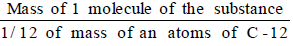Molecular Mass - Atoms and Molecules, Class 9, Science

# Molecular Mass - Atoms and Molecules, Class 9, Science - Notes - Class 9

 1 Crore+ students have signed up on EduRev. Have you?

Molecules:

A molecule is the smallest particle of an element or compound that has an independent existence. A molecule contains one or more than one atoms.

The molecules of elements contain atoms of only one kind.

The number of atoms in a molecule of an element is known as atomicity of the element. For example -The atomicity of the noble gases is 1, that of hydrogen, nitrogen, oxygen etc. is 2 each and of ozone is 3. Thus, noble gases, hydrogen and ozone are respectively monoatomic, diatomic and triatomic molecules.

Molecules of elements:

The molecules of an element contain two similar atoms chemically bonded together, for example, ozone gas has 3 oxygen atoms combined together, so ozone exists in the form of O3. A recently discovered form of carbon, called Buckminster fullerene has molecular formula C60.

Molecules of compounds:

The molecules of a compound contain two or more different types of atoms chemically bonded together. For example the molecule sulphur dioxide (SO2) contain one atom of sulphur chemically bonded with two atoms of oxygen.

Molecular mass and formula mass:

The molecular mass of a substance (an element or a compound) may be defined as the average relative mass of a molecule of the substance as compared with the mass of an atom of carbon (C-12 isotope) taken as 12 amu.

Molecular Mass =The molecular mass of a compound can be obtained by adding atomic masses of all the atoms present in the molecule of the compound. For example, molecular mass of CO2 is -

12 × 1 + 16 × 2 = 44 u

Gram Molecular Mass:

Gram molecular mass of a substance is defined as that much quantity of the substance whose mass expressed in grams is numerically equal to its molecular mass.

For example, The molecular mass of CO2 is 44 u, its gram molecular mass is 44g. Gram molecule mass of a substance is also known as the gram-molecular mass of the substance.

Formula Mass:

Formula mass of an ionic compound is obtained by adding atomic masses of all the atoms in a formula unit of the compound.

For example Formula mass of potassium chloride (KCl)

= Atomic mass of potassium atomic mass of chlorine

39 35.5 = 74.5

The document Molecular Mass - Atoms and Molecules, Class 9, Science - Notes - Class 9 is a part of Class 9 category.
All you need of Class 9 at this link: Class 9
 Use Code STAYHOME200 and get INR 200 additional OFF

Track your progress, build streaks, highlight & save important lessons and more!

,

,

,

,

,

,

,

,

,

,

,

,

,

,

,

,

,

,

,

,

,

,

,

,

,

,

,

;